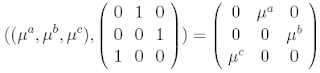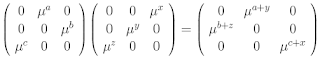The (wreath) product used in the theory of the field with one element is between the symmetric group $S_d$ and $d$ copies of the $n$-th roots of unity. For $\mu$ the primitive $n$-th root, an element of the product group is usually written likeand the multiplication is given by semidirect product
$(g_1 , \pi_1) \circ (g_2 , \pi_2) = (g_1 \cdot (\pi_1 g_2) , \pi_1 \pi_2)$ otherwise known as a 2-group composition. For this particular group, the semidirect product is given by ordinary matrix multiplicationThe examples assume that $d = 3$, but $n$ is fairly arbitrary. How does the three dimensional Fourier transform act on such phased circulants? We may decompose the result into a diagonal and a $1$-circulant, as inConsider the case where $\mu^a = \mu^c$, reducing the circulant towhere the diagonalisation of the ordinary $1$-circulant permutation is clear, if $\mu^a = \mu^b$. In order to be Hermitian, it is clear that the phases must satisfy $\mu^a = \pm 1$ and $\mu^b = \pm 1$. For example, when $(\mu^a , \mu^b)=(1,-1)$ then the two real eigenvalues for this operator are given by
$\lambda = \frac{1}{3} (1 - 4 \textrm{cos}(\frac{2 \pi k}{3})) \in -1, 1$
which are the values of $\mu^i$ as square roots of unity. Note that the Hermitian condition can only be satisfied here if at least two of the roots are the same, so this is the general situation for such phased operators. The natural generalisation is to replace $S_d$ with the braid group on $d$ strands, labelling strands with roots of unity.